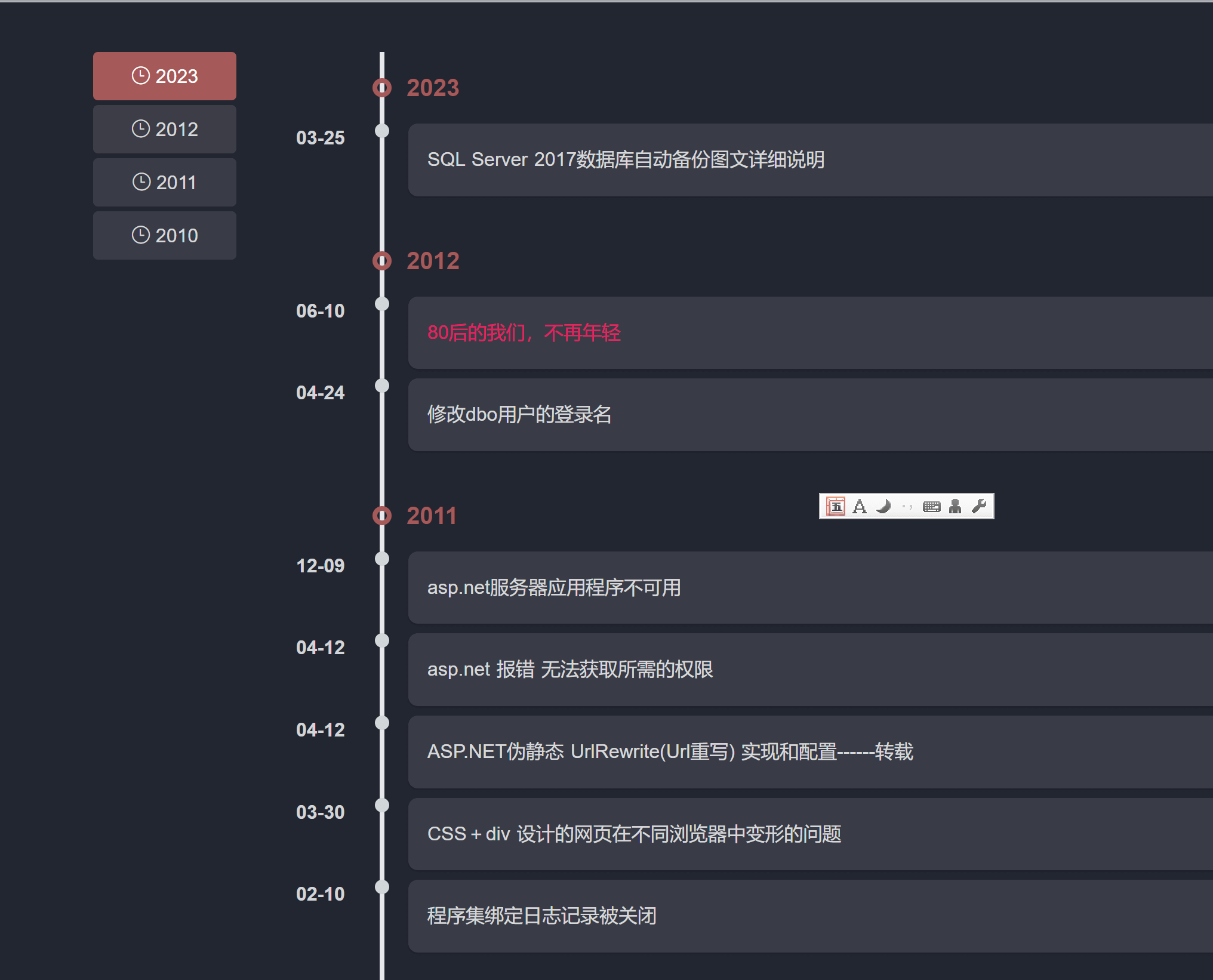# 这个BLOG模板样式能用开发标签实现吗？

johnnypro 发表于    阅读:107    回复:12

```<?php \$yearData = \MBlog::listBlogByYear(); ?>
<div>
共有 {{\$yearData['total']}} 篇博客
</div>
@foreach(\$yearData['records'] as \$year=>\$yearItem)
<div>
{{\$yearItem['year']}}年{{\$yearItem['count']}}篇
</div>
<div>
<ul>
@foreach(\$yearItem['records'] as \$b)
<li>
<a href="{{modstart_web_url('blog/'.\$b['id'])}}">
{{\$b['title']}}
</a>
</li>
@endforeach
</ul>
</div>
@endforeach```

`date('m-d',strtotime(\$b['postTime']))````<?php \$yearData = \MBlog::listBlogByYear(); ?>
<div>
共有 {{\$yearData['total']}} 篇博客
</div>
@foreach(\$yearData['records'] as \$year=>\$yearItem)
<div>
{{\$yearItem['year']}}年{{\$yearItem['count']}}篇
</div>
<div>
<ul>
<?php \$currentDay = null; ?>
@foreach(\$yearItem['records'] as \$b)
<?php \$d = date('Y-m-d',strtotime(\$b['postTime']); ?>
@if(!\$currentDay || \$currentDay!=\$d)
<?php \$currentDay = \$d; ?>
<li>
{{ \$d }}
</li>
@endif
<li>
<a href="{{modstart_web_url('blog/'.\$b['id'])}}">
{{\$b['title']}}
</a>
</li>
@endforeach
</ul>
</div>
@endforeach```

`<?php \$d = date('Y-m-d',strtotime(\$b['postTime']); ?>`

ＱＱ